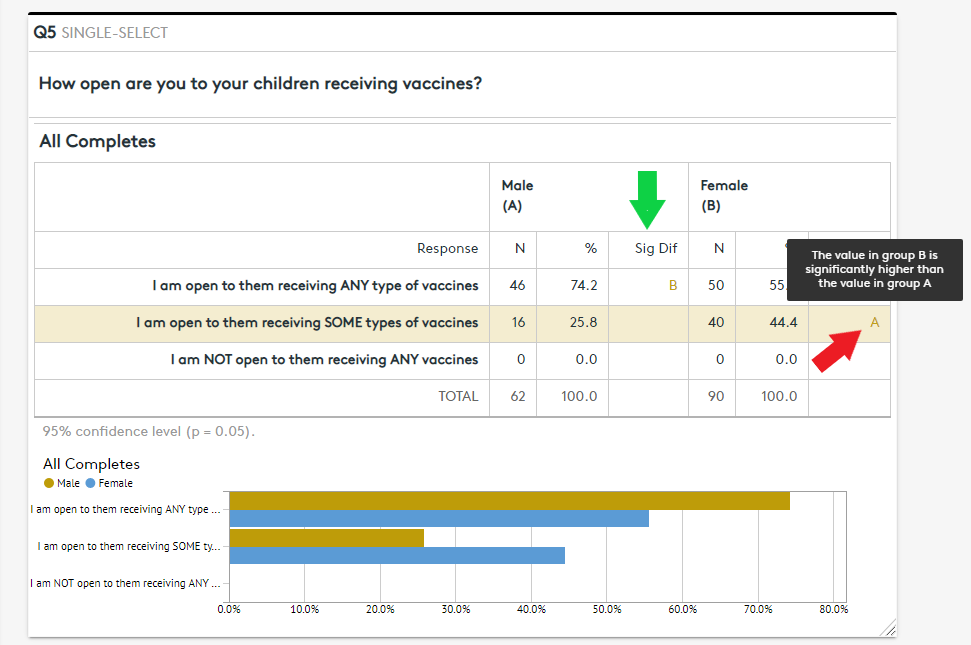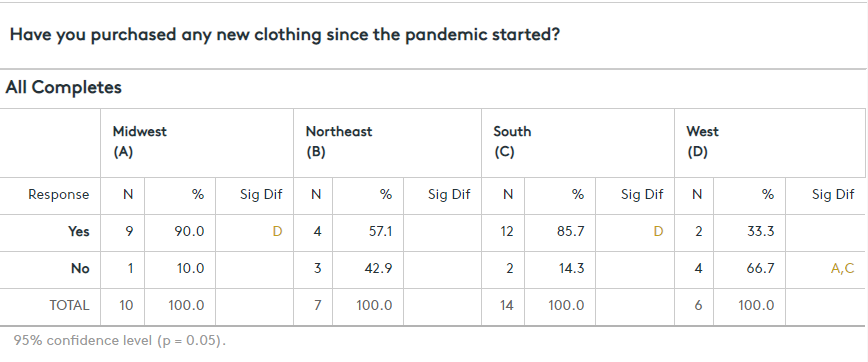Statistically significance indicators let you know when data in one column is reliably different from data in another column on the same row.  After cross-tabbing your questions and turning the statistical significance feature on, you can use statistical significance indicators to quickly identify groups in your data which are reliably and repeatedly different from another group answering the same questions. Once identified, you should weigh the importance of the results to determine if they are actionable or not.

Why should I use the Statistical Significance feature?
Turning on this feature will make it markedly easier to identify instances where one group’s answers are reliably different from another group’s answers.

How do I turn it on?

First, select a crosstab question to evaluate. Then, to turn on Statistical Significance, simply expand the Statistical Significance section on the left side of the results page and check the checkbox labeled “Show Statistical Significance”. You can leave the Confidence Level at 95% most of the time.

What will I see?
When you first turn on Statistical Significance (also known as Stat Testing) you will see new columns on your grid questions and cross tabbed questions labeled “Sig Diff”. On cross tabbed questions, within these columns, you will see gold letters matching the labels applied to each column in your grid. Hovering your mouse over one of the letters will provide you with a description.

Note: On grid questions, statistical significance calculations are currently unavailable. As such, you will not see statistical significance indicators in the “Sig Diff” columns on grids.What does "confidence level" mean?
The percentage of time that a statistical result would be correct if you took numerous random samples using the same audience targeting criteria. Confidence is often associated with assuredness, and the statistical meaning is closely related to this common usage of the term.

Which confidence-level value should I use?
The market research standard is 95% confidence level. However, you may want to increase/decrease this based on the size of your sample.  With very large sample sizes, you can increase the confidence level to quiet the noise in the results. With smaller sample sizes, you may want to decrease the confidence level in order to surface additional significance indicators.

How do I talk about statistical significance when I share the information I discovered in my survey?
We suggest using the phrase "reliably repeatable”. Using an example from the table below, one might say that, "When we look at the purchase behavior of US citizens in the Midwest and South regions during the pandemic, we can expect reliably repeatable higher-instances of them buying clothing compared to those in the West region."How many respondents are needed for the calculation?
The short answer is - 51 or more.  While we do provide significance indicators for questions with at least 31 responses, any question with 31-50 responses should be used for directional indication (is reliably higher than/is reliably lower than) and should not be talked about in terms of statistical significance.

Is the stat testing more accurate with more respondents?
Absolutely. In fact, we suppress the significance indicators on questions where the banner (question responses across the top) has fewer than 31 answers and we provide a cautionary warning for those questions with 50 or fewer responses.

The nerd-stuff...

What statistical significance formula does Express Surveys use?

NAME

Two Independent Samples % Test

INPUTS

p1           proportion sample1 (%/100)

p2           proportion sample2 (%/100)

n1           base size sample1

n2           base size sample2

FORMULA

diff = abs(p1 - p2)

ap = (p1*n1 + p2*n2) / (n1+n2)

aq = 1 - ap

se = sqrt(ap * aq / (1 - (1 / (n1+n2))) * (1/n1+1/n2))

Continuity Correction Factor ALWAYS applied

cf = -0.5 * (1/n1 + 1/n2)

z = (diff + cf) / se

TEST

if z > tinv(1-level, n1 + n2 -2), significant at level below